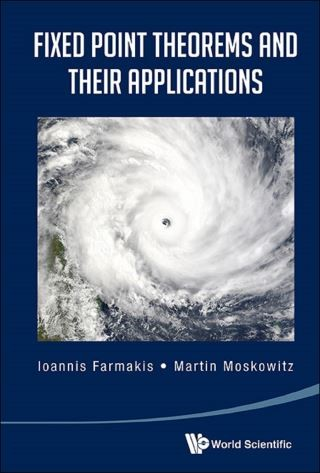## Fixed Point Theorems And Their Applications• Description
• Author
• Info
• Reviews

### Description

This is the only book that deals comprehensively with fixed point theorems throughout mathematics. Their importance is due, as the book demonstrates, to their wide applicability. Beyond the first chapter, each of the other seven can be read independently of the others so the reader has much flexibility to follow his/her own interests. The book is written for graduate students and professional mathematicians and could be of interest to physicists, economists and engineers.

Contents:
• Early Fixed Point Theorems
• Fixed Point Theorems in Analysis
• The Lefschetz Fixed Point Theorem
• Fixed Point Theorems in Geometry
• Fixed Points of Volume Preserving Maps
• Borel's Fixed Point Theorem in Algebraic Groups
• Miscellaneous Fixed Point Theorems
• A Fixed Point Theorem in Set Theory

Readership: Graduate students and professionals in analysis, approximation theory, algebra and geometry.
Key Features:
• Our book gives a complete treatment of the diverse and manifold use of fixed point theorems and their many applications throughout Mathematics and is another example of the unity within Mathematics
• As such it serves as a valuable resource for researchers in diverse fields of Mathematics
• It also serves as solid introduction for students to several subjects in modern Mathematics such as Functional Analysis, Topology, Differential Geometry, Dynamical Systems and Algebraic Groups